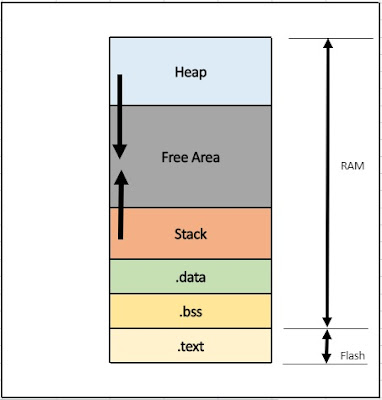# Memory layout of executable in embedded system

7

1. Structure of elf file
2. Highlights of linker file. How .bss, .txt, .stack and .heap sections are mapped into micro-controllers memory using linker file.

## 1. Introduction :Fig. 1 Memory layout of embedded system
When we compile c code finally we get an executable file (e.g. .elf file). Fig 1. shows the general memory layout of c program. You all are aware of general segments of memory, which are .txt, .bss, .data, stack and heap.

But have you ever thought how these segments are created and where are these segments actually resides in micro-controller? If yes then you are reading right article.

## 2. Linker File and Memory Sections

We all know object files (.o files). Linker's job is to combine all those object files and create a final executable file. Linker file defines size and memory location of each segments which are going to be load into micro-controller's memory. .bss, .data, .txt, stack and heap segments are loaded into micro-controllers memory and which memory to go defined in linker file.

Consider example of NXP S32K144 linker file as an example. There are two commands generally used in linker script, those are MEMORY and SECTION.

Before getting into details of MEMORY and SECTION commands lets have look at memory map of of S32K144

1. 4GB of address space as it is 32-bit controller.
2. 512 KB of P-Flash  memory addr:  0x0000  0000  to 0x0007   FFFF
3. 32KB of SRAM L   memory addr:  0x1FFF 8000  to 0x1FFF  FFFF
4. 28KB of SRAM U   memort addr:   0x2000  0000  to 0x2000   6FFF

MEMORY command is used to define size and location of a memory block. These memory blocks are nothing but partition of physical memory present in controller. Partition depends on memory map.  Linker script decides which part of memory to use and which should not be used.      In linker file of  S32K144, below sections are created.

MEMORY
{
/* Flash */
m_interrupts            (RX)  : ORIGIN = 0x00000000, LENGTH = 0x00000400
m_flash_config        (RX)  : ORIGIN = 0x00000400, LENGTH = 0x00000010
m_text                      (RX)  : ORIGIN = 0x00000410, LENGTH = 0x0007FBF0

/* SRAM_L */
m_data                     (RW)  : ORIGIN = 0x1FFF8000, LENGTH = 0x00008000

/* SRAM_U */
m_data_2                 (RW)  : ORIGIN = 0x20000000, LENGTH = 0x00007000
}

m_text memory block starts from 0x00000410 and its size is 510KB
m_data memory block starts from 0x1FFFF8000 and size if 32KB so which is nothing but SRAM L
m_data_2 memory block is nothing but SRAM U

SECTION command tells linker how to  map input sections into output sections. Now input sections are .data, .txt,.bss, stack and heap and output sections are m_data, m_data_2 and m_text.
below lines are parts of linker script of NXP S32k144 series, which highlights on memory segments.

.txt section , mapped to  m_text section, :
.text :
{
. = ALIGN(4);
*(.text)                   /* .text sections (code) */
*(.text*)                 /* .text* sections (code) */
*(.rodata)               /* .rodata sections (constants, strings, etc.) */
*(.rodata*)             /* .rodata* sections (constants, strings, etc.) */
*(.glue_7)              /* glue arm to thumb code */
*(.glue_7t)             /* glue thumb to arm code */
*(.eh_frame)
KEEP (*(.init))
KEEP (*(.fini))
. = ALIGN(4);
} > m_text

.bss section mapped to  m_data_2 section :
.bss :
{
/* This is used by the startup in order to initialize the .bss section. */
. = ALIGN(4);
__BSS_START = .;
__bss_start__ = .;
*(.bss)
*(.bss*)
*(COMMON)
. = ALIGN(4);
__bss_end__ = .;
__BSS_END = .;
} > m_data_2

.data section mapped to  m_data section :
.data : AT(__DATA_ROM)
{
. = ALIGN(4);
__DATA_RAM = .;
__data_start__ = .;      /* Create a global symbol at data start. */
*(.data)                     /* .data sections */
*(.data*)                 /* .data* sections */
KEEP(*(.jcr*))
. = ALIGN(4);
__data_end__ = .;        /* Define a global symbol at data end. */
} > m_data

.stack section mapped to  m_data_2
.stack :
{
. = ALIGN(8);
. += STACK_SIZE;
} > m_data_2

.heap section mapped to m_data_2
.heap :
{
. = ALIGN(8);
__end__ = .;
PROVIDE(end = .);
PROVIDE(_end = .);
PROVIDE(__end = .);
__HeapBase = .;
. += HEAP_SIZE;
__HeapLimit = .;
__heap_limit = .;
} > m_data_2

This is how in linker file memory sections are created and at time of linking phase linker will combine all .O files, based on linker script keep variables functions and code in particular memory section of micro-controller. .bss section of all .O files will be combined into m_data_2 segment.
Tags

1.Excellent article....but don't try to make it short...but it's really great

2.3.Excellent description.Thank you

4.Thanks for sharing

5.Thanks for giving clarity but i have a question:
From above we can see code section of a program will be in flash,then while executing the program will the code still remain in flash or shall it be moved to ram ??if moved to ram then to which place as there no structure is in place for the code.
6.7.